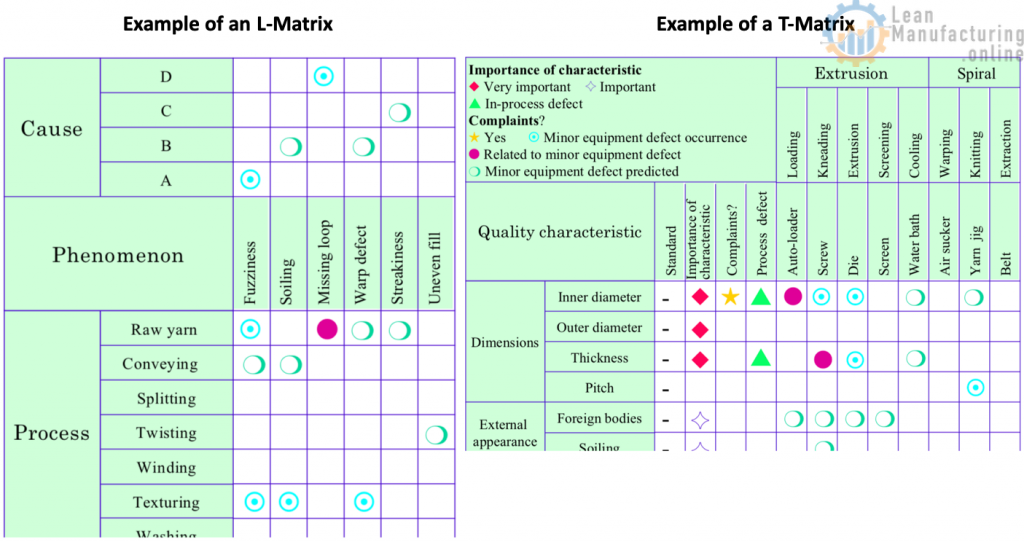Quality control needs to be based on facts rather than experience or intuition.

The original purpose of quality control was to reduce the number of product defects in mass production, and their chief cause was thought to be variability, so statistical tools were used to address the problem. Statistical tools used in quality control include control charts, histograms, Pareto diagrams, design of experiments, and sampling inspection. Non-statistical approaches are also used, in the form of cause-and-effect diagrams, QFD, FMEA and FTA, among others.

These methods focus on graphic representation of data likely to prove useful in solving the problem, and their advantage is that they make the problem visible. Control makes it possible to achieve a planned value for a given task. The basic approach to control is to repeat the following sequence: Plan→ Do→Check (inspect/diagnose)→ Act (repair/improve). This is called the “PDCA cycle” or the “control cycle”, see slide.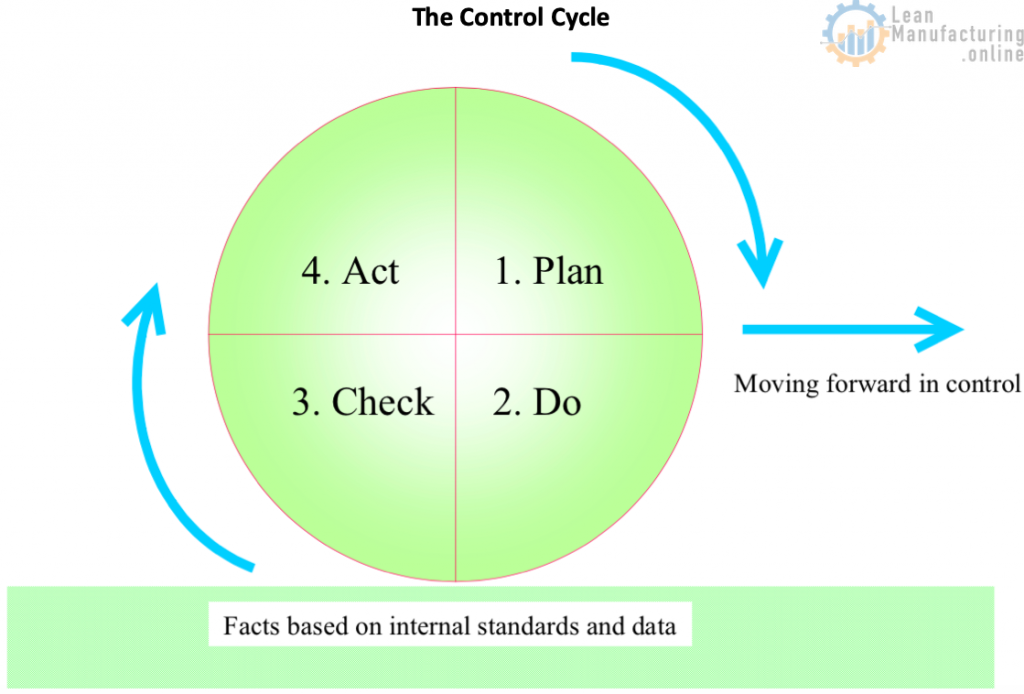## 1. Cause-and-effect diagrams

Also known as the fishbone diagram or Ishikawa diagram was developed by the Japanese quality guru Dr. Kaoru Ishikawa to express one of the basic principles of total quality management, that the desired product, target or other outcome is achieved not by directly controlling the results themselves but by controlling the processes that produce those results (since a process is a collection of causes leading to the results).

Cause-and-effect diagrams are sometimes called as “4-M Diagram” or “4-M Analysis” (Man, Machine, Material, Method). Nowadays it has been expanded to 6-M, adding Mother Nature (to analyze causes related to Environment) and Measurement (part of original Ishikawa diagram).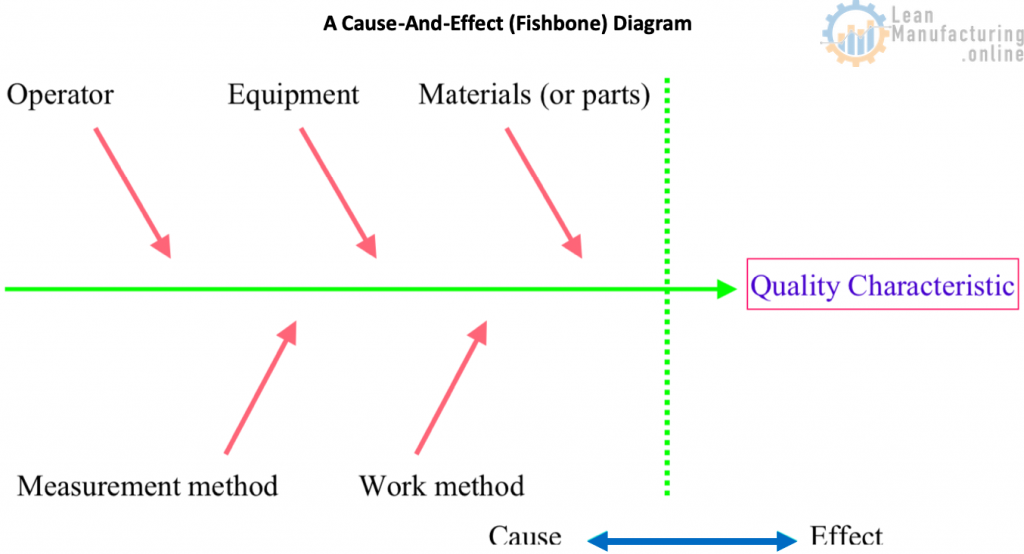## 2. Checksheets

Checksheets are printed lists or diagrams of information required for control purposes. Once dealt with, each item is ticked, making data-collection more efficient and transparent. Checksheets can be designed to investigate the distribution of process parameters, the occurrence or causes of product defects, or the location of equipment defects.

## 3. Histograms

Although the overall distribution pattern can be deduced from the data points in a frequency distribution table, the distribution can also be represented as a column graph, known as a histogram, see slide. Employed to reveal mean values and variation patterns, the histogram plays an important role as a process analysis technique for tasks such as checking for defectives by comparing product quality characteristics against standard values: – Frequency Distribution Tables – Normal Distribution – Standard Deviation## 4. Pareto Diagrams

A Pareto diagram is a kind of frequency distribution graph. Imagine a set of data relating to equipment failures, rework, errors, complaints and other losses. The data specifies the financial cost of each loss, how often it occurs, what percentage of the total loss it accounts for, and so on. The losses may also be classified by their causes or the situations in which they appear. If this data is represented in the form of a bar chart with the values in descending order, it will be obvious at a glance which category has the most failures, defects, etc. Plotting the cumulative total for each category on the resulting histogram will produce a Pareto diagram see slide. A Pareto diagram enables causes to be ranked, and thus prioritized and tackled more effectively.## 5. Scatter Diagrams

With a set of data all of the same type, the overall distribution pattern can be found by a method such as plotting the frequency distribution. However, if there are two corresponding sets of data (such as height and weight), and the task is to find the relationship between the two, a scatter diagram, or scattergram, is used, see slide. The relationship between two corresponding sets of data such as temperature and yield, or between workpiece dimensions before and after processing, is called a correlation. A correlation can be either positive or negative.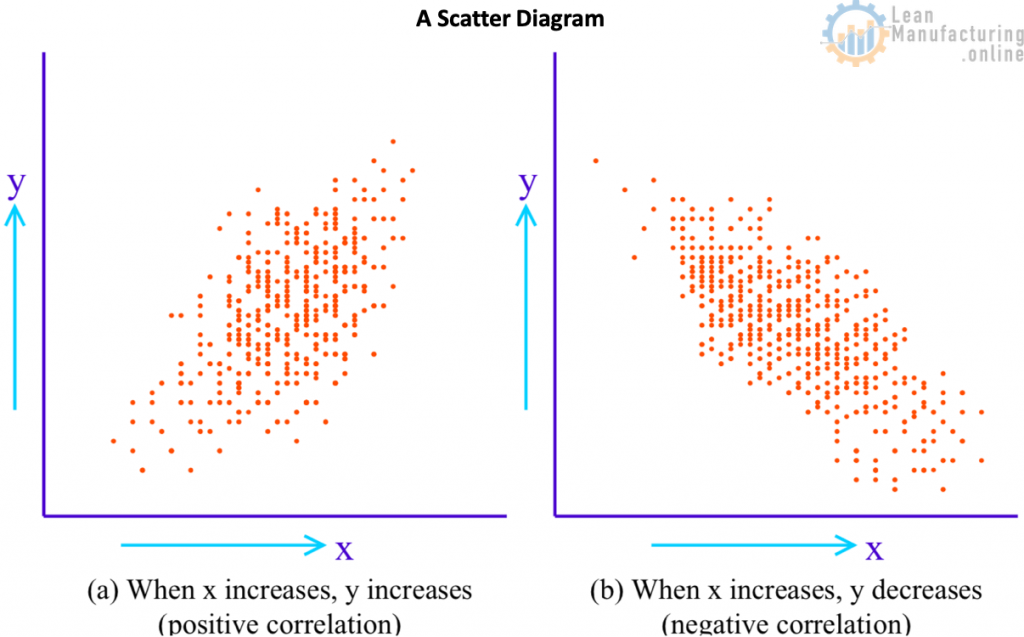## 6. Control Charts

A control chart is a line graph on which control limit lines are plotted in order to find out if a process is in a stable condition, or in order to keep it so. Control charts can be used for attributes (discrete data that, by its nature, can only be expressed as positive integers, such as numbers of people and incidences of failure), or for variables (continuous data such as length, weight, time or temperature). – X − R Control Chart – p Control Chart – np Control Chart – Control Limit lines Any graphical representation without control lines is simply a graph, rather than a control chart. A control chart has a central line (CL), an upper control limit (UCL) and a lower control limit (LCL), collectively known as control lines, see slide.## 7. Graphs and charts

These are the basic tools of statistical control. The purpose of using statistical control techniques is to clarify the severity of problems, and identify their causes. Although problems cannot be solved by graphs and charts alone, they can certainly help in tracking down their causes. For this reason, graphs and charts are an essential part of the problem-solving toolkit.

• Bar Charts
• Pie Charts
• Line Graphs
• As mentioned before, line graphs show how a quantity changes, especially over time. They are also known as function graphs, broken-line graphs, or time-series charts. In contrast to bar charts, which are characterized by the fact that they compare quantities at a fixed point in time, the chief feature of line graphs is that they provide an intuitive, overall insight into how a phenomenon varies over time. It is therefore important to emphasize the points rather than the lines when drawing them, see slide.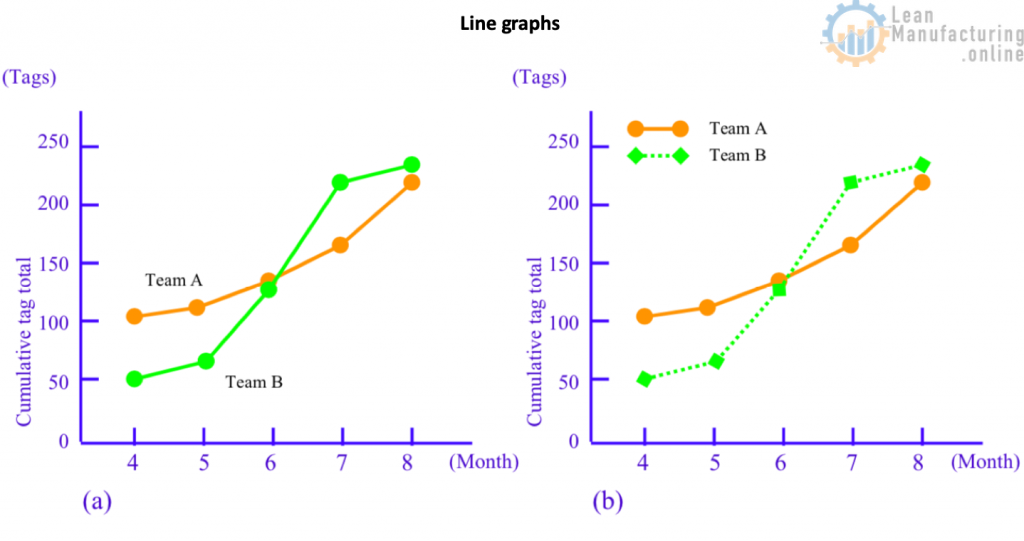• Compound Bar Charts
• Although we have now established that the three basic types of graph are bar charts, pie charts and line graphs, their applications are many and varied. Some of the more commonly used varieties include compound bar charts (also known as stacked bar charts or strip charts; see slide), pictographs, area graphs, volume charts and combination graphs.